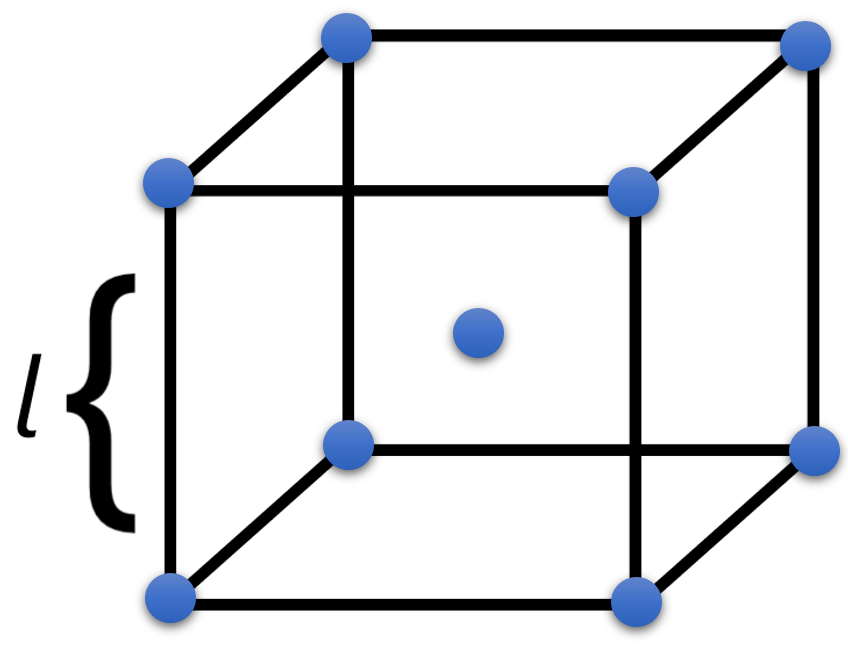# Problem: An unknown metal is found to have a density of 7.8748 g/cm3 and to crystallize in a body-centered cubic lattice. The edge of the unit cell is found to be 0.28864 nm .Calculate the atomic mass of the metal.

###### FREE Expert Solution

We are asked to determine the atomic mass of a metal with a body-centered cubic lattice

Recall that a body-centered cubic lattice has 8 atoms in its 8 corners and 1 atom at the center:To solve for the atomic mass, we will follow the steps:

STEP 1: Find the volume of the unit cell

STEP 2: Find the mass of the unit cell

STEP 3: Calculate the atomic mass

93% (369 ratings)###### Problem Details

An unknown metal is found to have a density of 7.8748 g/cm3 and to crystallize in a body-centered cubic lattice. The edge of the unit cell is found to be 0.28864 nm .

Calculate the atomic mass of the metal.